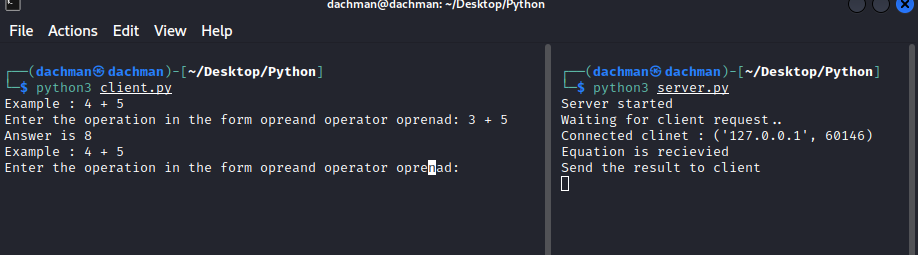# Simple Calculator in Python Socket Programming

• Last Updated : 26 Aug, 2022

In this article, we are going to know how to make a simple calculator in Python socket programming.

Prerequisite: Socket Programming in Python.

First, we will understand the basics of Python socket programming. Socket programming is used to set up a communication channel between two nodes on a network. It provides Inter connected communication (IPC). The network can be a logical, local network and physical network to connect external network. In this example, we will write local socket programming code in Python.

## Socket Module

Python has a remarkably large library of functional modules from which we are going to use the Socket module. This module provides us to make networking programs in Python. In this module, we will be focused on connect method and socket method.

• socket() method helps to make socket INET stream.
• connect() method helps to connect the server.

### Methode used

Syntax: socket.socket(socket.AF_INET, socket.SOCK_STREAM)

Parameters:

• socket.AF_INET: It refers to the address-family ipv4.
• socket.SOCK_STREAM: It refers to connection-oriented TCP protocol.

Syntax: socket.connect(IP Address of the Server,Port Number)

Parameters:

• IP Address: IP address of  the server.
• Port Number: Port Number on which we have to connect.

Note: To establish the connection between the client and server we have to run the Python server program first and then run the Python client program otherwise if we run the client program first it gives a connection error.

## Python Client

First, we will write client-side code. A client is a user or machine which generates a request to the server in return it gets the response from the server side. To perform these tasks we have to know two things which are the Port Number and IP Address of the Server.

In this case, We will use localhost IP which is 127.0.0.1  and the port number is “8080”.

## Python3

 `# Import socket module``import` `socket` `# In this Line we define our local host``# address with port number``SERVER ``=` `"127.0.0.1"``PORT ``=` `8080``# Making a socket instance``client ``=` `socket.socket(socket.AF_INET,``                       ``socket.SOCK_STREAM)``# connect to the server``client.connect((SERVER, PORT))``# Running a infinite loop``while` `True``:``    ``print``(``"Example : 4 + 5"``)``    ``# here we get the input from the user``    ``inp ``=` `input``("Enter the operation ``in` `\``the form opreand operator oprenad: ")``    ``# If user wants to terminate``    ``# the server connection he can type Over``    ``if` `inp ``=``=` `"Over"``:``        ``break``    ``# Here we send the user input``    ``# to server socket by send Method``    ``client.send(inp.encode())` `    ``# Here we received output from the server socket``    ``answer ``=` `client.recv(``1024``)``    ``print``(``"Answer is "``+``answer.decode())``    ``print``(``"Type 'Over' to terminate"``)` `client.close()`

## Python Server

The server-side is a machine on which all the operations are executed as requested by the client-side, we will perform addition, subtraction, multiplication, and division operation on the server. To do so the server received input from a client and performs the basic operation by itself. when the result is ready Python server then sends the output to the client machine.

## Python3

 `# Import socket module``import` `socket` `# Here we use localhost ip address``# and port number``LOCALHOST ``=` `"127.0.0.1"``PORT ``=` `8080``# calling server socket method``server ``=` `socket.socket(socket.AF_INET,``                       ``socket.SOCK_STREAM)``server.bind((LOCALHOST, PORT))``server.listen(``1``)``print``(``"Server started"``)``print``(``"Waiting for client request.."``)``# Here server socket is ready for``# get input from the user``clientConnection, clientAddress ``=` `server.accept()``print``(``"Connected client :"``, clientAddress)``msg ``=` `''``# Running infinite loop``while` `True``:``    ``data ``=` `clientConnection.recv(``1024``)``    ``msg ``=` `data.decode()``    ``if` `msg ``=``=` `'Over'``:``        ``print``(``"Connection is Over"``)``        ``break` `    ``print``(``"Equation is recievied"``)``    ``result ``=` `0``    ``operation_list ``=` `msg.split()``    ``oprnd1 ``=` `operation_list[``0``]``    ``operation ``=` `operation_list[``1``]``    ``oprnd2 ``=` `operation_list[``2``]` `    ``# here we change str to int converstion``    ``num1 ``=` `int``(oprnd1)``    ``num2 ``=` `int``(oprnd2)``    ``# Here we are perform  basic arithmetic operation``    ``if` `operation ``=``=` `"+"``:``        ``result ``=` `num1 ``+` `num2``    ``elif` `operation ``=``=` `"-"``:` `        ``result ``=` `num1 ``-` `num2``    ``elif` `operation ``=``=` `"/"``:``        ``result ``=` `num1 ``/` `num2``    ``elif` `operation ``=``=` `"*"``:``        ``result ``=` `num1 ``*` `num2` `    ``print``(``"Send the result to client"``)``    ``# Here we change int to string and``    ``# after encode send the output to client``    ``output ``=` `str``(result)``    ``clientConnection.send(output.encode())``clientConnection.close()`

Output:My Personal Notes arrow_drop_up